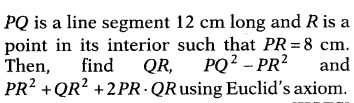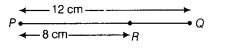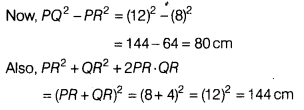# PQ is a line segment 12 cm long and R is a point in its interior such that PR = 8 cmGiven, PQ = 12 cm and PR = 8 cmHere, point R lies in the interior of PQ.
So, PR + QR = PQ
8+QR = 12
On subtracting 8 from both sides, we get
8 + QR-8 = 12-8 [by axiom 3]
QR=4 cm